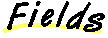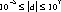`Packages  This Package  Prev  Next  Index  `

# §1.7 Class Float

```public  final  class  java.lang.Float
extends  java.lang.Number  (I-§1.11)
{
// Fields
public final static float MAX_VALUE;	§1.7.1
public final static float MIN_VALUE;	§1.7.2
public final static float NaN;	§1.7.3
public final static float NEGATIVE_INFINITY;	§1.7.4
public final static float POSITIVE_INFINITY;	§1.7.5

// Constructors
public Float(double  value);	§1.7.6
public Float(float  value);	§1.7.7
public Float(String  s);	§1.7.8

// Methods
public double doubleValue();	§1.7.9
public boolean equals(Object  obj);	§1.7.10
public static int floatToIntBits(float  value);	§1.7.11
public float floatValue();	§1.7.12
public int hashCode();	§1.7.13
public static float intBitsToFloat(int  bits);	§1.7.14
public int intValue();	§1.7.15
public boolean isInfinite();	§1.7.16
public static boolean isInfinite(float  v);	§1.7.17
public boolean isNaN();	§1.7.18
public static boolean isNaN(float  v);	§1.7.19
public long longValue();	§1.7.20
public String toString();	§1.7.21
public static String toString(float  f);	§1.7.22
public static Float valueOf(String  s);	§1.7.23
}
```
This class wraps a value of primitive type float in an object. An object of type Float contains a single field whose type is float.

In addition, this class provides a number of methods for converting a float to a String and a String to a float, as well as other constants and methods useful when dealing with a float.

##### MAX_VALUE

```public final static float MAX_VALUE ``` ``` = 3.40282346638528860e+38. ```
The largest positive finite value of type float.

### MIN_VALUE

```public final static float MIN_VALUE ``` ``` = 1.40129846432481707e-45 ```
The smallest positive value of type float.

### NaN

```public final static float NaN = 0.0f/0.0f ```
The Not-a-Number value of type float.

### NEGATIVE_INFINITY

```public final static float NEGATIVE_INFINITY ``` ``` = -1.0f/0.0f ```
The negative infinity of type float.

### POSITIVE_INFINITY

```public final static float POSITIVE_INFINITY ``` ``` = 1.0f/0.0f ```
The positive infinity of type float.

##### Float

```public Float(double value) ```
Constructs a newly allocated Float object that represents the argument converted to type float.
Parameters:
```value - ```the value to be represented by the Float

### Float

```public Float(float value) ```
Constructs a newly allocated Float object that represents the primitive float argument.
Parameters:
```value - ```the value to be represented by the Float

### Float

```public Float(String s) ``` ```throws NumberFormatException ```
Constructs a newly allocated Float object that represents the floating-point value of type float represented by the string. The string is converted to a float value as if by the valueOf method (I-§1.7.23).

Parameters:
```s - ```a string to be converted to a Float
Throws
NumberFormatException (I-§1.41)
If the string does not contain a parsable number.

##### doubleValue

```public double doubleValue() ```
The float value represented by this object is converted to type double and the result of the conversion is returned.
Overrides:
doubleValue in class Number (I-§1.11.1).

### equals

```public boolean equals(Object obj) ```
The result is true if and only if the argument is not null and is a Float object that represents a float that has the identical bit pattern to the bit pattern of the float represented by this object.
Note that in most cases, for two instances of class Float, f1 and f2, the value of f1.equals(f2) is true if and only if
f1.floatValue() == f2.floatValue()
also has the value true. However, there are two exceptions:
Returns:
true if the objects are the same; false otherwise.
floatToIntBits (I-§1.7.11).
Overrides:
equals in class Object (I-§1.12.3).

### floatToIntBits

```public static int floatToIntBits(float value) ```
The result is a representation of the floating-point argument according to the IEEE 754 floating-point "single precision" bit layout.
Bit 31 represents the sign of the floating-point number. Bits 30-23 represent the exponent. Bits 22-0 represent the significand (sometimes called the mantissa) of the floating-point number.
If the argument is positive infinity, the result is 0x7f800000.
If the argument is negative infinity, the result is 0xff800000.
If the argument is NaN, the result is 0x7fc00000.
Parameters:
```value - ```a floating point number
Returns:
the bits that represent the floating point number.

### floatValue

```public float floatValue() ```
Returns:
The float value represented by this object is returned.
Overrides:
floatValue in class Number (I-§1.11.2).

### hashCode

```public int hashCode() ```
Returns:
a hash code value for this object.
Overrides:
hashCode in class Object (I-§1.12.6).

### intBitsToFloat

```public static float intBitsToFloat(int bits) ```
The argument is considered to be a representation of a floating-point value according to the IEEE 754 floating-point "single precision" bit layout. That floating-point value is returned as the result.
If the argument is 0x7f800000, the result is positive infinity.
If the argument is 0xff800000, the result is negative infinity.
If the argument is any value in the range 0x7f800001 through 0x7f8fffff or in the range 0xff800001 through 0xff8fffff, the result is NaN. All IEEE 754 NaN values are, in effect, lumped together by the Java language into a single value.
Parameters:
```bits - ```an integer
Returns:
the single format floating-point value with the same bit pattern.

### intValue

```public int intValue() ```
Returns:
The float value represented by this object is converted to type int and the result of the conversion is returned.
Overrides:
intValue in class Number (I-§1.11.3).

### isInfinite

```public boolean isInfinite() ```
Returns:
true if the value value represented by this object is positive infinity (I-§1.7.5) or negative infinity(I-§1.7.4); false otherwise

### isInfinite

```public static boolean isInfinite(float v) ```
Parameters:
```v - ```the value to be tested
Returns:
true if the argument is positive infinity (I-§1.7.5) or negative infinity(I-§1.7.4); false otherwise

### isNaN

```public boolean isNaN() ```
Returns:
true if the value value represented by this object is NaN (I-§1.7.3); false otherwise

### isNaN

```public static boolean isNaN(float v) ```
Returns:
true if the argument is NaN (I-§1.7.3); false otherwise
Parameters:
```v - ```the value to be tested

### longValue

```public long longValue() ```
Returns:
The float value represented by this object is converted to type long and the result of the conversion is returned.
Overrides:
longValue in class Number (I-§1.11.4).

### toString

```public String toString() ```
The primitive float value represented by this object is converted to a String exactly as if by the method toString of one argument (I-§1.7.22).

Returns:
a String representation of this object.
Overrides:
toString in class Object (I-§1.12.9).

### toString

```public static String toString(float f) ```
Creates a string representation of the float argument.
The values NaN, NEGATIVE_INFINITY, POSITIVE_INFINITY, -0.0, and +0.0 are represented by the strings "NaN", "-Infinity", "Infinity", "-0.0" and "0.0", respectively.
If d is in the range, then it is converted to a String in the style [-]ddd.ddd. Otherwise, it is converted to a string in the style [-]m.ddddE±±±±xx.
There is always a minimum of one digit after the decimal point. The number of digits is the minimum needed to uniquely distinguish the argument value from adjacent values of type float.
Parameters:
```d - ```the float to be converted
Returns:
a string representation of the argument.

### valueOf

```public static Float valueOf(String s) ``` ```throws NumberFormatException ```
Parses a string into a Float.
Parameters:
```s - ```the string to be parsed
Returns:
a newly constructed Float initialized to the value represented by the String argument.
Throws
NumberFormatException (I-§1.41)
If the string does not contain a parsable number.

`Packages  This Package  Prev  Next  Index`
Java API Document (HTML generated by dkramer on April 22, 1996)